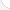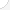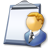Biological scientistsDescription: Biological scientists study living things and their environment. They do research, develop new medicines, increase crop amounts, and improve the environment. Some study specialty areas such as viruses, ocean life, plant life, or animal life. They may work in company, college, or government labs, or as high school biology teachers.Source: Bureau of Labor Statistics, U.S. Department of Labor, Occupational Outlook Handbook, 2008-09 Edition at http://www.bls.gov/OCO/Complete Job Profile: http://www.bls.gov/oco/ocos047.htmSalary: \$50,001 or more per yearComments:

There are 51 math topics Biological scientists need to know.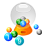Basic Math / Algebra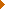FractionsDecimalsRatio and ProportionPercentCustomary MeasurementMetric MeasurementMeasurement ConversionBasic ProbabilityBasic StatisticsStatistical GraphingPowers and RootsOther Number BasesNegative NumbersScientific NotationBasic Problem Solving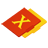First-Year AlgebraUsing FormulasLinear EquationsLinear InequalitiesOperations with PolynomialsFactoring PolynomialsRational ExpressionsCoordinate Graphing 2DLinear SystemsAlgebraic Representation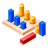GeometryBasic TerminologyAngle MeasurementTriangle InequalitiesSimilarityGeometric MeanPythagorean TheoremRight Triangle TrigonometryAreaVolume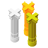Second-Year Algebra / TrigonometryFunctionsVariationLogarithmsMatricesAdvanced ProbabilityAdvanced StatisticsTrigonometric/Circular FunctionsPolar Coordinates/GraphsVectors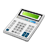Other TopicsCalculus and Higher MathBasic Calculator UseScientific Calculator UseComputer UseGroup Problem SolvingMental MathInductive/Deductive ReasoningMath CommunicationsMathematical Modeling